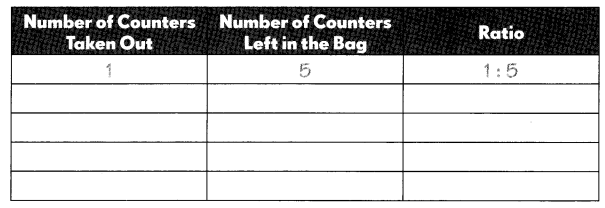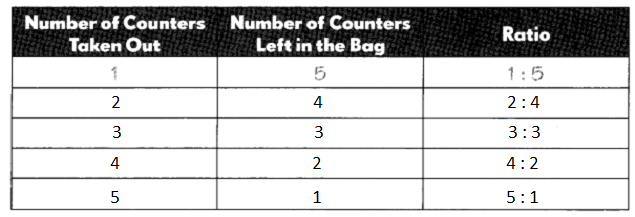# Math in Focus Grade 5 Chapter 7 Practice 1 Answer Key Finding Ratio

Practice the problems of Math in Focus Grade 5 Workbook Answer Key Chapter 7 Practice 1 Finding Ratio to score better marks in the exam.

## Math in Focus Grade 5 Chapter 7 Practice 1 Answer Key Finding Ratio

The table shows the number of points each student scored in a math game.

Find the total number of points the students scored.

Question 1.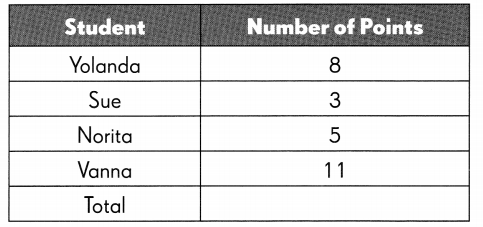Answer: Total number of points the students scored are 27.

Explanation:
Given,
Yolanda has 8,
Sue has 3,
Norita has 5,
Vanna has 11,
So by adding 8, 3, 5 and 11 we get 27.

Complete the table to show the ratios.

Question 2.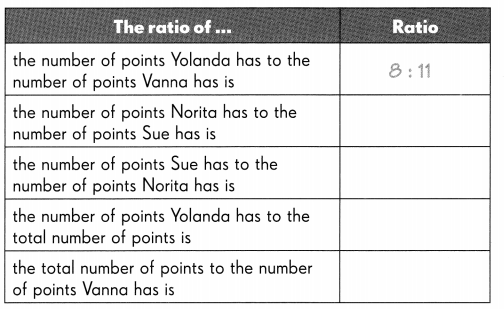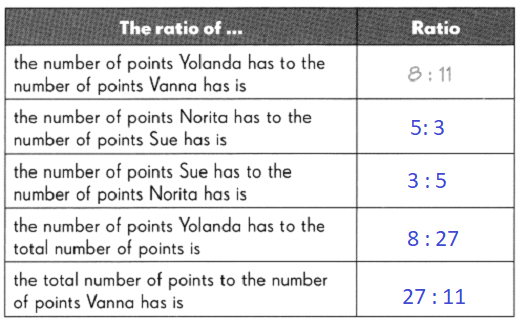Complete.

Mr. Gonzales put some pencils into bundles of 10. He gave 4 bundles to Charlie and 9 bundles to Lisa.

Question 3.
The ratio of the number of pencils Charlie has to the number of pencils
Lisa has is : ___ : _____
Explanation:
Given,
Mr. Gonzales put some pencils into bundles of 10,
He gave 4 bundles to Charlie,
And 9 bundles to Lisa,
So the ratio of the number of pencils Charlie to the number of pencils Lisa has is 4 : 9.

Question 4.
The ratio of the number of pencils Lisa has to the number of pencils Charlie
has is : ___ : _____
Explanation:
Given,
Mr. Gonzales put some pencils into bundles of 10,
He gave 4 bundles to Charlie,
And 9 bundles to Lisa,
So the ratio of number of pencils Lisa to the number of pencils Charlie has is 9 : 4.

Question 5.
The ratio of the number of pencils Lisa has to the total number of pencils is
____ : _____

Explanation:
Given,
Mr. Gonzales put some pencils into bundles of 10,
He gave 4 bundles to Charlie,
And 9 bundles to Lisa,
So by adding 9 with 4 we get thirteen as total,
The ratio of number of pencils Lisa to the total number of pencils is 9 : 13.

This table shows the amount of milk and spring water that four families drink in a week.

Find the total amount of milk and water that they drink.

Question 6.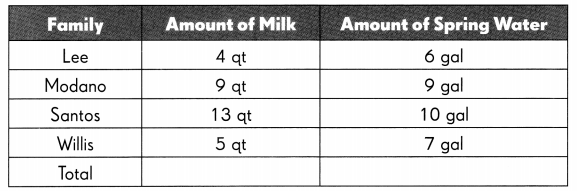The amount of milk is 31 quantity
And the amount of spring water is 32 quantity.
Explanation:
By adding each of the familiy’s quantity of milk such as 4, 9, 13 and 5 we get 31,
By adding each familiy’s quantity of spring water such as 6, 9, 10 and 7 we get 32.

Use the above table to fill in the blanks.

Example
The ratio of the amount of water the Santos family drinks to the amount of water the Modano family drinks is 10:9

Question 7.
The ratio of the amount of milk the Modano family drinks to the amount of milk the Willis family drinks is ____

Use the table on page 234 to fill in the blanks.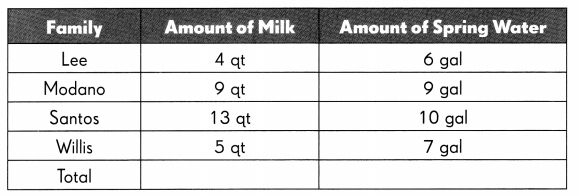Question 8.
The ratio of the amount of water the Willis family drinks to the amount of water the Lee family drinks is ____

When writing two quantities as a ratio, the quantities must be in the same unit. The ratio itself however has no units.Question 9.
The ratio of the total amount of milk to the amount of milk the Modano family drinks is ____________

Question 10.
The ratio of the amount of water the Santos family drinks to the total amount of water is ____.

Complete.

Question 11.
The ratio of the length of A to the length of C is ________ : _____.

Question 12.
The ratio of the length of C to the length of B is _______ : ____.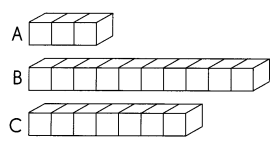Question 13.
The ratio of the length of A to the total length of A, B, and C is ____: ____

Complete.

Question 14.
The ratio of the length of R to the length of P is ________ : _____.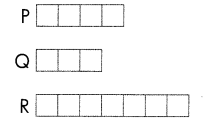Question 15.
The ratio of the length of P to the length of Q is ________ : ___.

Question 16.
The ratio of the length of P to the total length of P,Q and R is _____ : _____.

Draw models to show each ratio.

Question 17.
5 : 9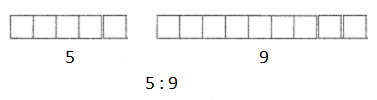Question 18.
12 : 7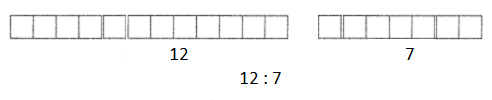Solve.

Question 19.
Grandma gave $15 to Linda and Dianne. Linda got$7.

a. How much money did Dianne get?
Given,
Grandma gave $15 to Linda and Dianne Linda got$7
By subtracting $7 from$15 we get $8, So Dianne got$8.

b. Find the ratio of the amount of money Linda got to the amount of money Dianne got from Grandma.
Explanation:
The ratio of the amount of money Linda and Diaanne got from Grandma is 7 : 8.

Solve.

Question 20.
Amelia has 25 postcards. She gives 8 away.

a. How many postcards does she have left?
Explanation:
Given,
Amelia has 25 postcards
She gives away 8 of them,
By subtracting 8 from 25 we get 17,
So there are 17 postcards left with Amelia.

b. Find the ratio of the number of postcards Amelia has left to the number of postcards she had at first.
Explanation:
Amelia has 17 number of postcards left after giving away 8 of them from 25 postacrds she had at first.

Question 21.
Clark has two 16-ounce cans of corn. He uses 1 8 ounces of it to make a corn soup and the rest to make a casserole.

a. How many ounces of corn did he use to make the casserole?
Explanation:
Given,
Clark has two 16-ounces cans of corn,
2 x 16 = 32 ounces of corn
He uses 18 ounces of corn to make a corn soup,
32 – 18 = 14
Clark has 14 ounces of corn to make a casserole.

b. What is the ratio of the amount of corn Clark used to make the casserole to the amount of corn he had at first?
Explanation:
The ratio amount of corn Clark used to make the casserole to the amount of corn he had at first is 14 : 32.

Question 22.
In a supermarket bin, the number of packages of red peppers to the number of packages of green peppers is in the ratio 8:13. The peppers are said in 2-pound packages.

a. What is the least possible weight of red peppers in the bin?
Explanation:
Given,
The number of packages of red peppers is 8,
The peppers are sold in 2 pound packages,
By multiplying 8 with 2 we get 16,
8 x 2 = 16
The least possible weight of red peppers in the bin is 16 pounds.

b. What is the least possible weight of green peppers in the bin?
Explanation:
Given,
The number of packages of green peppers is 13,
The peppers are sold in 2 pound packages,
By multiplying 13 with 2 we get 26,
13 x 2 = 16
The least possible weight of red peppers in the bin is 26 pounds.

Leanne put 6 counters into a bag. She took out some counters from the bag but not all of them.

Find the ratio of the number of counters taken out of the bag to the number of the counters left in the bag. Make a list of all possible ratios using the table.

Question 23.